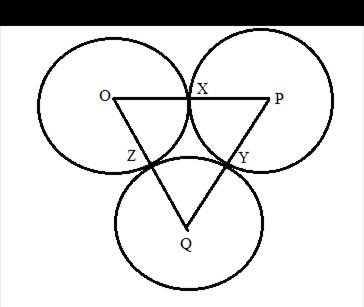Chapter 6.3, Problem 40EElementary Geometry For College St...

7th Edition
Alexander + 2 others
ISBN: 9781337614085

Solutions

Chapter
SectionElementary Geometry For College St...

7th Edition
Alexander + 2 others
ISBN: 9781337614085
Textbook Problem

Circles O, P, and Q are tangent (as shown) at points X, Y, and Z. Being as specific as possible, explain what type of triangle Δ P Q O is if:a) O X = 2 , P Y = 3 , Q Z = 1 b) O X = 2 , P Y = 3 , Q Z = 2To determine

(a)

To explain:

What type of triangle ΔPQO is if:

OX=2,PY=3,QZ=1

Explanation

Given that, circles O, P, and Q are tangent (as shown) at points X, Y, and Z.

Then the value of OX=2,PY=3,QZ=1.

The diagrammatic representation is given below,

To find the measures of the sides of the triangle ΔPQO is given below,

OP=OX+PXPQ=PY+QYOQ=QZ+OZ

We know that OX=OZ,PX=PY,QY=QZ since radius of the circle is same for all points on the circle

To determine

(b)

To find:

To explain what type of triangle ΔPQO is if:

OX=2,PY=3,QZ=2

Still sussing out bartleby?

Check out a sample textbook solution.

See a sample solution

The Solution to Your Study Problems

Bartleby provides explanations to thousands of textbook problems written by our experts, many with advanced degrees!

Get Started

In Exercises 516, evaluate the given quantity. log1616

Finite Mathematics and Applied Calculus (MindTap Course List)

In Exercises 5-8, solve for x. 3x2x224x=0

Calculus: An Applied Approach (MindTap Course List)

Use the given graph of f to sketch the graph of f1. 29.

Single Variable Calculus: Early Transcendentals, Volume I

In Exercises 722, evaluate the expression. 21. 161/4 81/3

Applied Calculus for the Managerial, Life, and Social Sciences: A Brief Approach

Find f. f(x) = 20x3 12x2 + 6x

Single Variable Calculus: Early Transcendentals

y=4x2+1 defines y implicitly as a function of x.

Study Guide for Stewart's Single Variable Calculus: Early Transcendentals, 8th